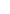## Electrical Fundamentals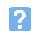1. Which one of the following gives the units of electric charge?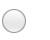a) Amperesb) micronsc) Coulmbsd) electrons2. A current of 62.5 mA is the same as:a) 0.00625 Ab) 0.0625 Ac) 0.625 Ad) 6.25 A3. A frequency of 3.75 MHz is the same as:a) 37.5 kHzb) 375 kHzc) 3,750 kHzd) 37,500 kHz4. Which one of the following statements is TRUE?a) Electrons and protons are both neutralb) Electrons carry a negative charge but protons carry no chargec) Electrons carry a positive charge and protons carry an equal negative charged) Protons carry a positive charge and electrons carry an equal negative charge5. Potential difference is measured in:a) Ampsb) Joulesc) Ohmsd) Volts6. Which one of the following metals is the best conductor of electric current?a) aluminiumb) copperc) silverd) steel7. The force between two charges is proportional to:a) the sum of the two chargesb) the ratio of the two chargec) the product of the two chargesd) the difference of the two charges.8. The relative permittivity of free space is:a) zerob) 1c) between 1 and 10d) between 10 and 1009. Which of the graphs below (showing flux density plotted against magnetising force) is typical of steel?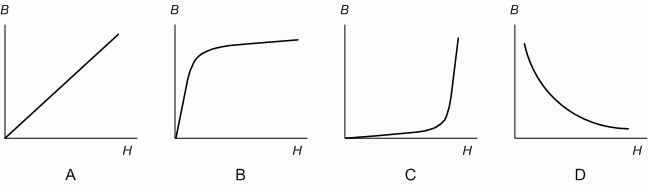a) Ab) Bc) Cd) D10. Which of the diagrams below shows the magnetic field around a coil carrying an electric current?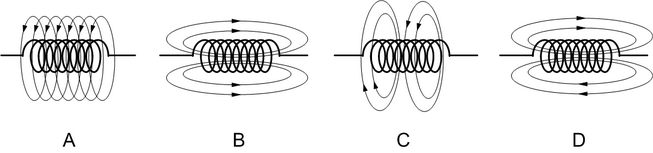a) Ab) Bc) Cd) D11. Which one of the components shown below is a toggle switch?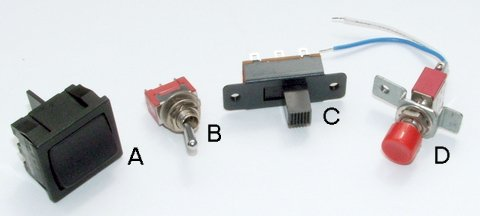a) Ab) Bc) Cd) D12. What do the symbols marked X and Y represent?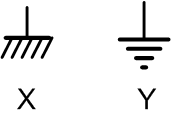a) X represents an earth connection, Y represents a chassis connectionb) X represents a chassis connection, Y represents an earth connectionc) X represents a battery connection, Y represents a power supply connectiond) X represents a power supply connection, Y represents a battery connection.13. In the circuit shown below, what type of switch is S1?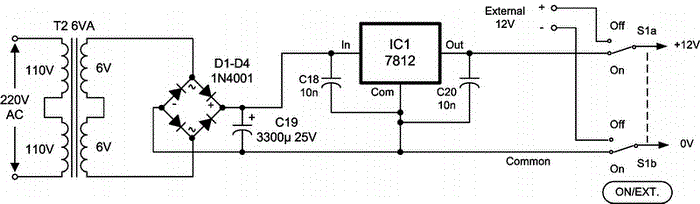a) DPDTb) DPSTc) SPDTd) SPST14. A signal has a frequency of 400 Hz. Which one of the following gives its periodic time?a) 2.5 msb) 25 msc) 250 msd) 400 ms15. A capacitor has a marked value of 47,000 pF. This is the same as:a) 0.0047 uFb) 0.047 uFc) 4.7 nFd) 470 nF.
This is more feedback!
This is the feedback!